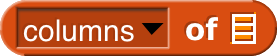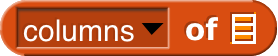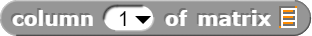# How Does Matrix Multiplication Work?

I want to intuitively know how it works. For example, say I want this:

I want to know how that works, step by step.

Solution:

Are you asking how to program it, how to do it on paper, what it means?

Sorry. I should have made it more clear what exactly I wanted. I just edited it.

Umm. Okay, I'll try...

You're running a factory that makes products out of metal. Each product requires so many grams of each kind of metal, so you have a table like this:

             steel       brass       aluminum

widget          87           0             15


Each kind of metal has a wholesale price (how much you have to pay) and a retail price (how much you charge customers) per gram:

                      wholesale         retail

steel                         23            42
brass                         14            20
aluminum                       7            12


You want to know the wholesale and retail materials cost for each product.

So, a widget is 87 grams of steel, 0 of brass, and 15 of aluminum. Its wholesale materials cost is therefore 87×23 for the steel, plus 0×14 for brass, plus 15×7 for aluminum. You'll do a total of four sums-of-products like that for wholesale and retail materials costs of the two things you manufacture (widgets and wadgets).

Does all that make sense? Well, instead of calling those tables "tables," leave out the captions and call them "matrices." Then the product of those two matrices is a two-by-two matrix whose rows are widget/wadget and whose columns are wholesale/retail.

This idea of combining two tables, in which the columns of the first one correspond to the rows of the second one, turns out to be useful in all sorts of contexts. Here's another one: You want to represent a transformation of a picture. So the rows and columns are both coordinates of points. If the picture is two-dimensional, you get a 2×2 matrix; if three-dimensional, 3×3. Taking 2-D for simplicity, the matrix

0 -1
1  0


represents a rotation by 90° clockwise around the origin, because the original point's distance to the right of the origin (its x coordinate) turns into a distance below the origin (a negative y coordinate), while the original point's distance above the origin turns into a distance to the right of the origin. If you represent a particular point as a one-row matrix (x y) and multiply that matrix by the one above, the result is a one-row matrix representing the coordinates after rotation. The transformation of doubling the height and width of a figure would be

2 0
0 2


and if you multiply the rotation one by this one, you get the transformation matrix for rotating and then doubling.

Does that help? If not, try to ask a more specific question.

I think I might be able to make a more intuitive one...

Yeah, the one in the APL library is complicated because it generalizes the idea to allow scalar operations other than + and ×, and to be careful about things like > 2 dimensions.

The one useful trick is to transpose the second matrix, which you can do without the APL library using, one of the options in the LENGTH pulldown.

Thanks for thetip! I'm now making a matrix library here.

Oh, just transpose it once, and then you can MAP over the result.

Are you talking about theblock?

Yes.

I'm constantly resaving, so just reload it often. Currently I have a MAP inside another MAP (in the scripts, not in a block), do you know if that could be used for the (matrix * matrix) block?

I know, that's why I graciously didn't comment on your first version of *. ;~)

You're making it more complicated than necessary, because you don't really trust MAP to do its job. You don't have to mess with indices. Nested MAPs will take care of combining every row with every column:

You write dot product; remember it has to compute a sum of products. (Its domain is vectors; its range is plain numbers.)

The first one.

Isn't that all elegant?

Ok. Just refresh and see.

Edit: Is this right?:

Yup!

I just solutioned your post, see it at the top. (There is no solution button in this category, so I just put a quote to the solution.)

cool.